# 成都美食数据分析

## 1.1美食数据获取

①run.py

②settings.py

③spider.py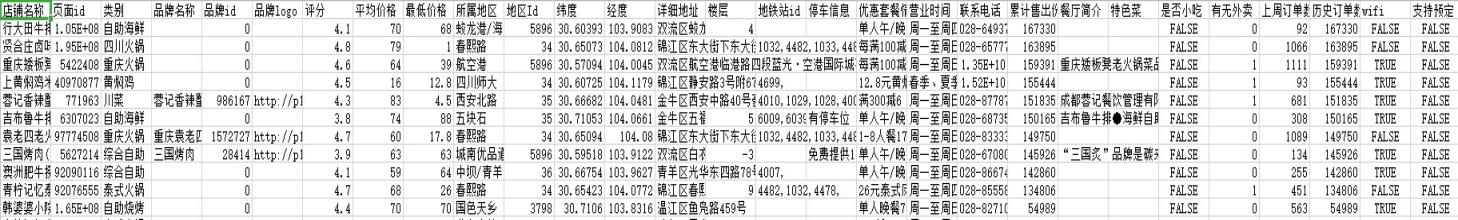limit:每页的店铺数量（我自定义的是30）
offset:翻页参数

## 1.2美食数据处理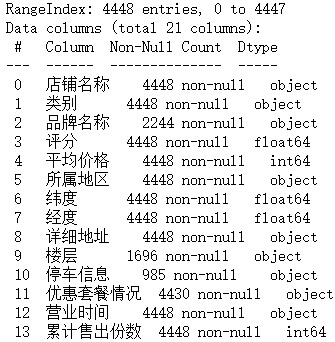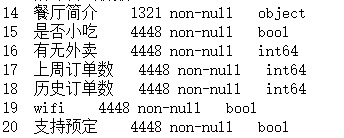df['区'] = df['详细地址'].str.findall('(.*?区)').str.get(0)## 1.3 美食数据可视化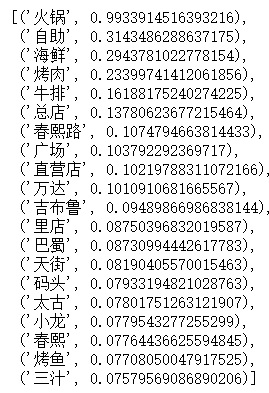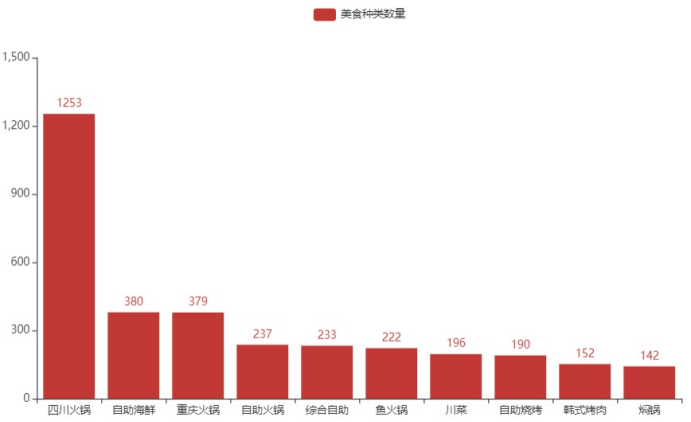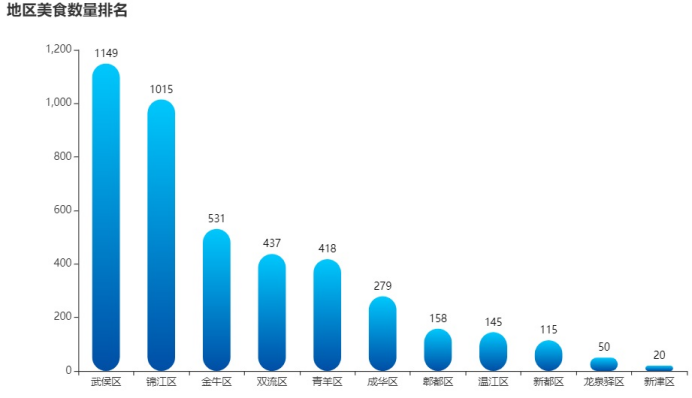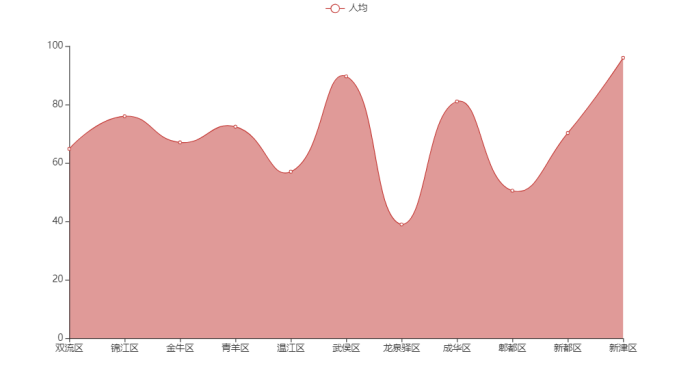pyecharts 模块中的 Geo 地理坐标系组件用于地图的绘制，支持在地理坐标系上绘制散点图,利用geo 函数画出将上述的餐厅数量在成都地图上的分布，可见大部分餐厅都集中在成都的中心区域，而周围区域的餐厅数量较少，且很分散。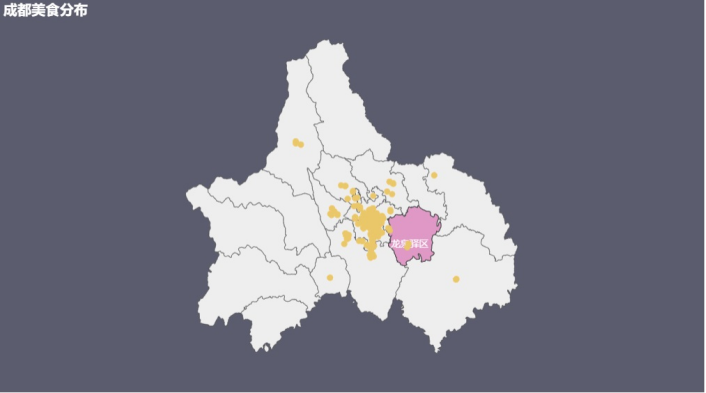# 成都租房数据分析

## 2.2 租房数据预处理

jieba.analyse.extract_tags
data.isnull().sum()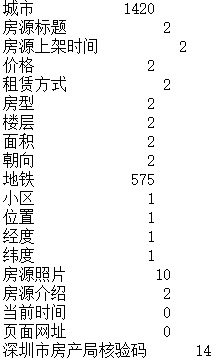#指定删除某列的缺失值
df2 = df.dropna(subset=['价格','租赁方式','房型','面积','朝向','位置'])
df2.isnull().sum()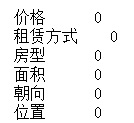• “面积”属性
“面积”属性的原数据里有m²，而需要的只有面积的数字信息，所以剔除m²，并将数据转换成整数型数据。
df2['面积'] = df2['面积'].str.replace('㎡', '').astype(dtype='int')

• “房型”属性
“房型”属性数据格式是xx室xx厅xx卫，它里面包含着室、厅、卫三个重要的数据属性，我们将这三个属性提取出来作为新属性。
df2[['室','厅','卫']] = df2['房型'].str.extract(r'(\d+)室(\d+)厅(\d+)卫')
df2['室'] = df2['室'].astype(float)
df2['厅'] = df2['厅'].astype(float)
df2['卫'] = df2['卫'].astype(float)

• ”价格”属性
“价格”属性格式杂乱无章，选择用正则方法提取我们需要的价格数字部分。
df2['价格'] = df2['价格'].map(lambda str: re.findall(r'\d+', str)).astype(dtype='int')

• “朝向”属性
该属性我们只需要朝向的方位，不需要前面的“朝向：”，所以直接将“朝向：”字段替换成空。
df2['朝向'] = df2['朝向'].str.replace('朝向：', '')

• “位置”属性
“位置”属性里面包含着很重要的一项——区域，众所周知区域这一因素对房租的影响很大，不同区的房租价格之间差距很大，这里用for循环将字符串中的区域属性提取出来。
word=list(df2['位置'])     #字符串转列表
l = len(word)
alist = []
for i in range(l):
word[i] = word[i][2:4]
alist.append(word[i])
df2['区'] = alist
df2.drop(['位置'], axis=1, inplace=True)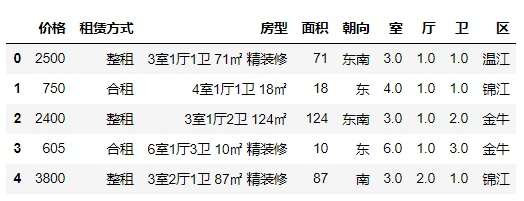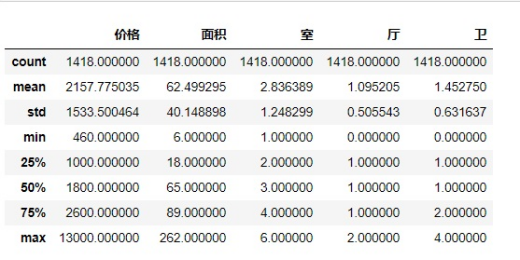• 哑变量处理
将非数值型数据转化为数值型数据，对类目型的特征因子化，对“朝向”，“租赁方式”，“区”这几个属性进行处理，做这步处理的原因在于许多算法模型建模需要输入的特征值都要为数值型，所以对于非数值型的数据值我们应当进行特征因子化。
df2_direction = pd.get_dummies(df2['朝向'],prefix= '朝向')
df2_zuling = pd.get_dummies(df2['租赁方式'],prefix= '租赁方式')
df2_area = pd.get_dummies(df2['区'],prefix= '区域')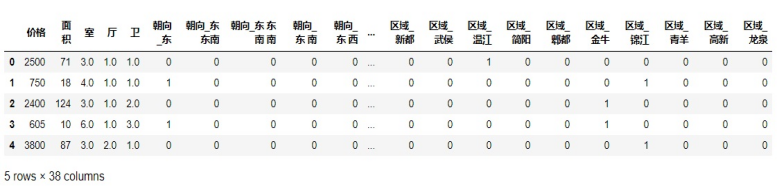from sklearn.preprocessing import StandardScaler
std_y = StandardScaler()
y_train = std_y.fit_transform(y_train.values.reshape(-1, 1))
y_test = std_y.fit_transform(y_test.values.reshape(-1, 1))


## 2.3 预测租房价格模型构造

（1）算法简介

（2）模型构造

1.从sklearn库中引入线性回归模型
from sklearn.linear_model import LinearRegression
2.线性回归模型训练
linear = LinearRegression()
lr_model = linear.fit(x_train,y_train)
3.将斜率、截距打印出来
print(lr_model.intercept_, model.coef_)

4.打印出模型的回归系数
pd.DataFrame({"columns":list(x_train.columns),"coef":list(lr_model.coef_.T)})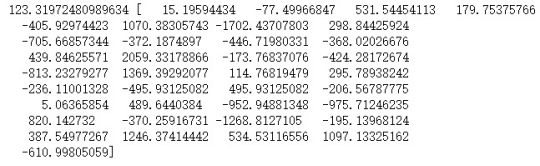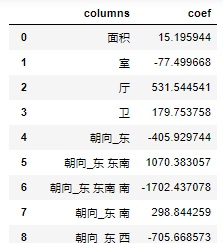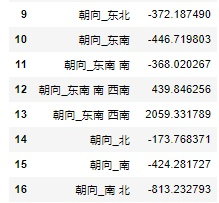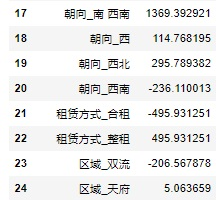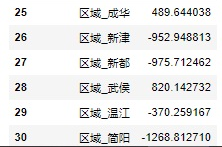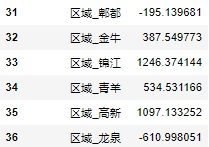k近邻分类(KNN)算法模型

（1）算法简介
k近邻法是一种基本分类与回归方法，是监督学习算法，可用于回归。该算法实现过程为存在一个样本数据集合，该样本数据集的属性已知，且其中每个数据都已知所属的分类。而对不知道分类的待分类数据，将待分类数据的每个属性属性与样本集中数据对应的属性比较，后算法提取样本中最相似的对象的分类标签，通常情况下我们只选择样本数据集中前k个最相似的对象数据，k一般是不大于20的整数，根据选择的这k个数据的属性，判断未分类数据的类型。
（2）模型构造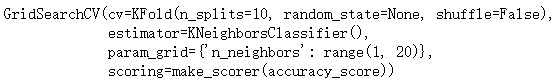scoring_fnc=make_scorer(accuracy_score)
kfold=KFold(n_splits=10)
pram_grid={'n_neighbors': range(1,20)}
clf=GridSearchCV(knn, pram_grid, scoring_fnc, cv=kfold)

clf.fit(x_train, y_train.astype('int'))


（1）算法简介
Keras是一个高层神经网络库，它核心的数据结构是模型，模型是一种组织网络层的方式。Keras中主要的Sequential模型是一系列的网络层按照顺序构成的栈。

（2）模型构造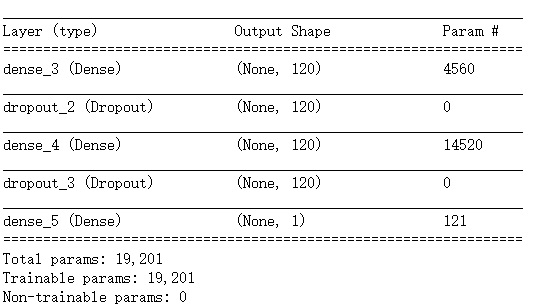1. 优化器（optimizer）：这里采用的是现有优化器字符串标识符rmsprop。

2. 损失函数（loss）：模型试图最小化的目标函数，它是平均绝对误差（meanabsolute error(MAE )，MAE是预测值与目标值之间差值的绝对值然后求平均，MAE损失比起MSE对于局外点更鲁棒。

3. 评估标准 metrics：MAE得分

## 2.4 模型性能评估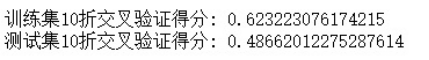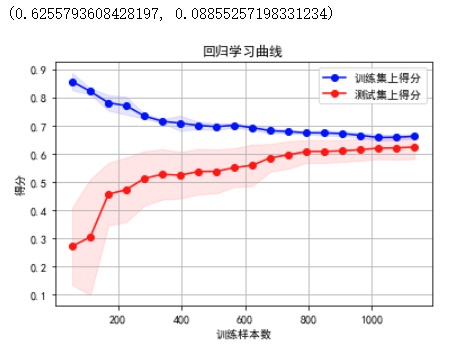Knn模型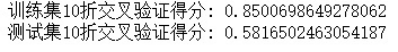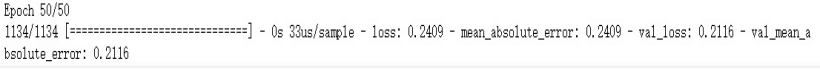# 总结与改进

## 3.2改进方面

①数据集

“房源介绍”属性中还包含有是否可以随时入住、楼层是高楼层还是低楼层、车位、用水、用电、燃气、采暖的情况，这些都是影响房租价格的因素，后期改进可以将它们的信息提取出来用。

②网络搜索平台多样化

③选择的模型

*欢迎大家一起唠嗑玩耍，一起共同进步！!03-13304
07-29665
04-23168
11-09358
12-133904
12-1744
10-152万+
08-192930
05-281337
07-111万+
10-27119
11-2519
08-27138
05-27681
11-01389
03-271749点击重新获取扫码支付余额充值